# 1 Exploratory Data Analysis

“Exploratory data analysis is detective work” [Tukey, 1977, p.2]. This package enables the user to use graphical tools to find ‘quantitative indications’ enabling a better understanding of the data at hand. “As all all detective stories remind us, many of the circumstances surrounding acrime are accidental or misleading. Equally, many of the indications to be discerned in bodies of data are accidental or misleading [Tukey, 1977, p.3].” The solution is to compare many different graphical tools with the goal to find an agreement or to generate an hypothesis and then to confirm it with statistical methods. This package serves as a starting point.

## 1.1 Synoptic Overview

``````library(DataVisualizations)
data("Lsun3D")
Pixelmatrix(Lsun3D\$Data)``````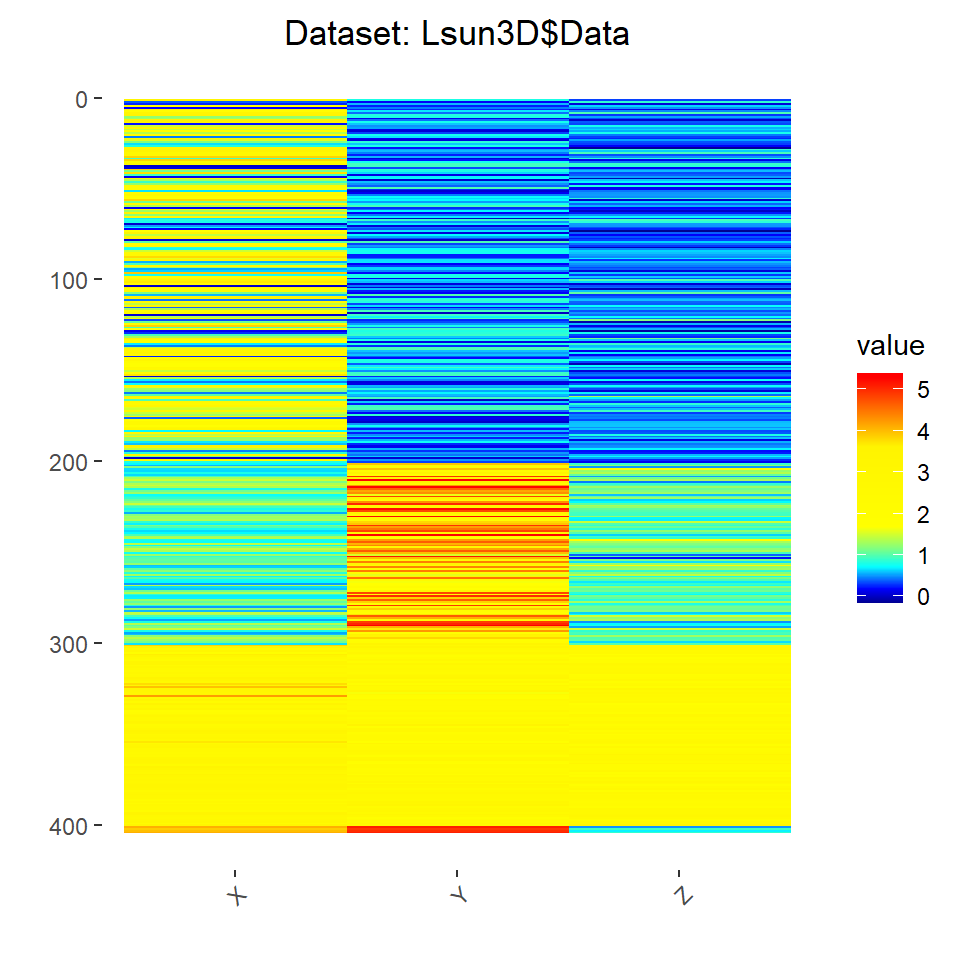## 1.2 Distribution Analysis

“A scientifically sound procedure for the identification and analysis of empirical distributions is a comparison to a known theoretic distribution. The quantile/quantile plot (QQ-plot) allows comparing an empirical distribution to a known distribution [Michael, 1983]. Here, in 100 quantiles the model of a Gaussian distribution is compared to the data, and a straight line confirms a good data fit of the model. The Gaussian distribution is the canonical starting point for such a comparison[…]

[t]he precise form, i.e., the type, nature and parameters of the formal model of the probability density function (pdf) is the […] goal of [Distribution] analysis. Usually, this is performed using kernel density estimators. The simplest of such a density estimation is the histogram. However, histograms are often misleading and require critical parameters such as the width of the bin [Keating and Scott, 1999]. A specially designed density estimation, which has been successfully proved in many practical applications is the “Pareto Density Estimation” (PDE). PDE consists of a kernel density estimator representing the relative likelihood of a given continuous random data [Ultsch, 2005]. PDE has been shown to be particularly suitable for the discovery of structures in continuous data hinting at the presence of distinct groups of data and particularly suitable for the discovery of mixtures of Gaussians [Ultsch, 2005]. The parameters of the kernels are auto-adopted to the date using an information theoretic optimum on skewed distributions [Ultsch, Thrun, Hansen-Goos, and Lötsch, 2015].” [Thrun/Ultsch 2018].

``````library(DataVisualizations)
data(MTY)
InspectVariable(MTY,'MTY')``````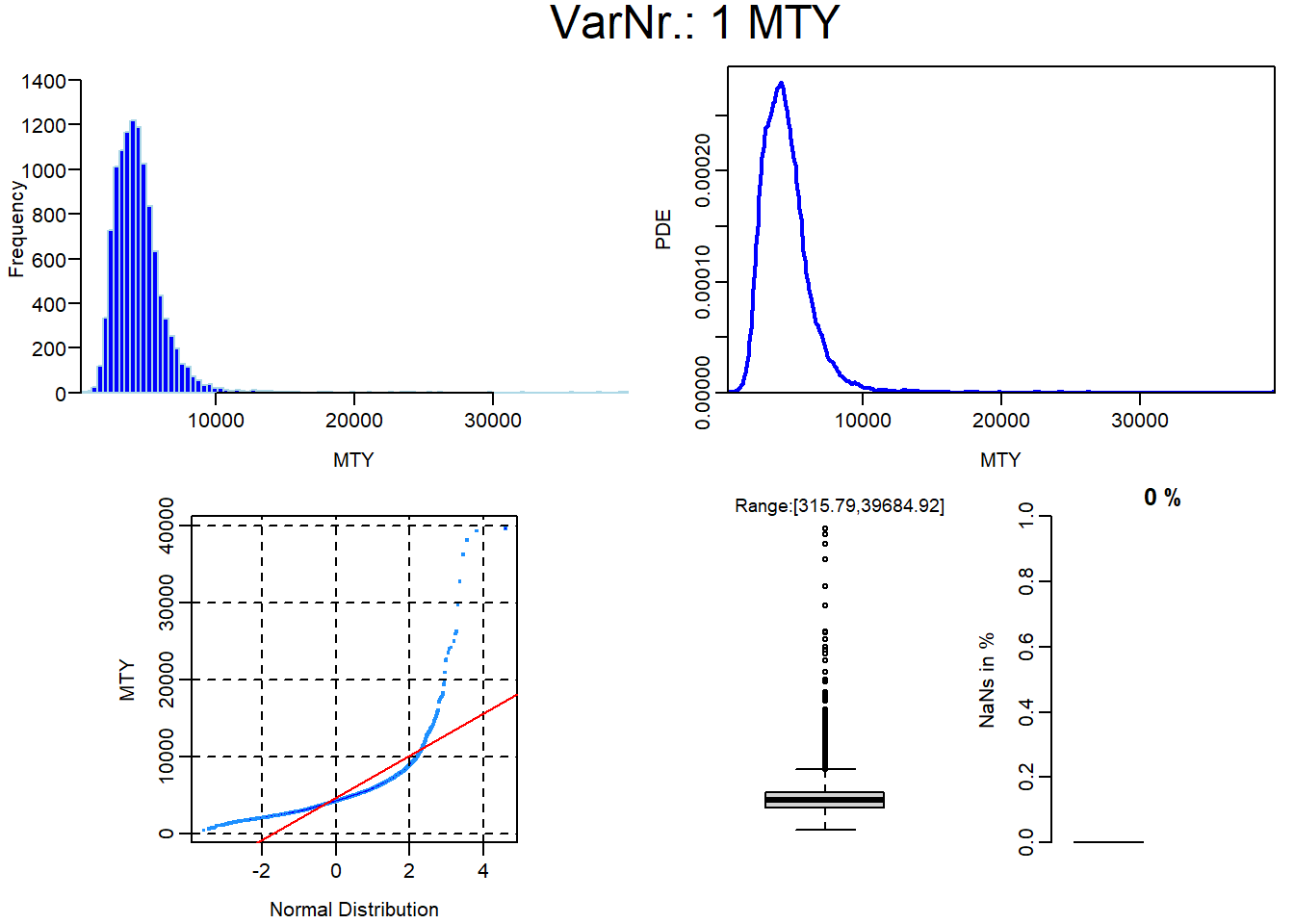## 1.3 Mirrored Density Plots (MD-plots)

A clear model behind density estimation can outperform conventional visualization approaches. MD Plot combines the syntax of ggplot2 with Pareto density estimation and additional functionality usefull from the Data Scientist’s point of view. The approach is published in [Thrun et al., 2020]. A detailed description of the usage and functionality can be found in https://md-plot.readthedocs.io/en/latest/index.html .

The MD plot is also available in Python https://pypi.org/project/md-plot/

All dependencies have to be installed so that the MDplot can be used:

``install.packages("DataVisualizations",dependencies = TRUE)``

Here, one feature is bi-modal the other one has a large range of values.

``````library(DataVisualizations)
library(ggplot2)
data(ITS)
data(MTY)
library(vioplot)
Data=cbind(ITS,MTY)

#MDplot(Data,Scaling = "Robust")+ggtitle('"Shape-Invariant" Normalization')

#Data is now capped
#Data[Data[,2]>6000,2]=6000
MDplot(Data)+ylim(0,6000)+ggtitle('Two Features with MTY Capped')``````
``## Warning: Removed 1614 rows containing non-finite values (`stat_pd_edensity()`).``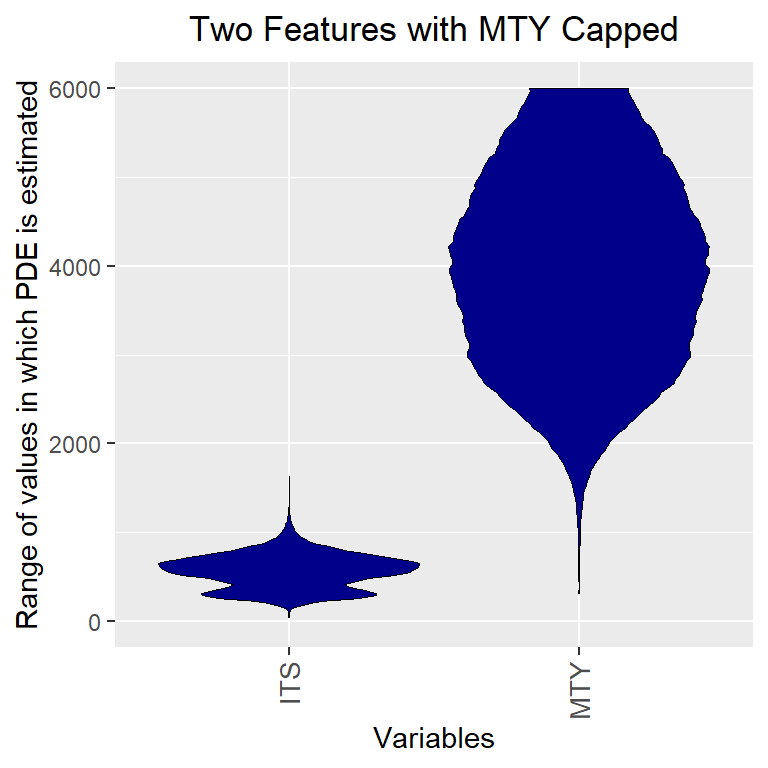``boxplot(Data,main='Two Features with MTY Capped')``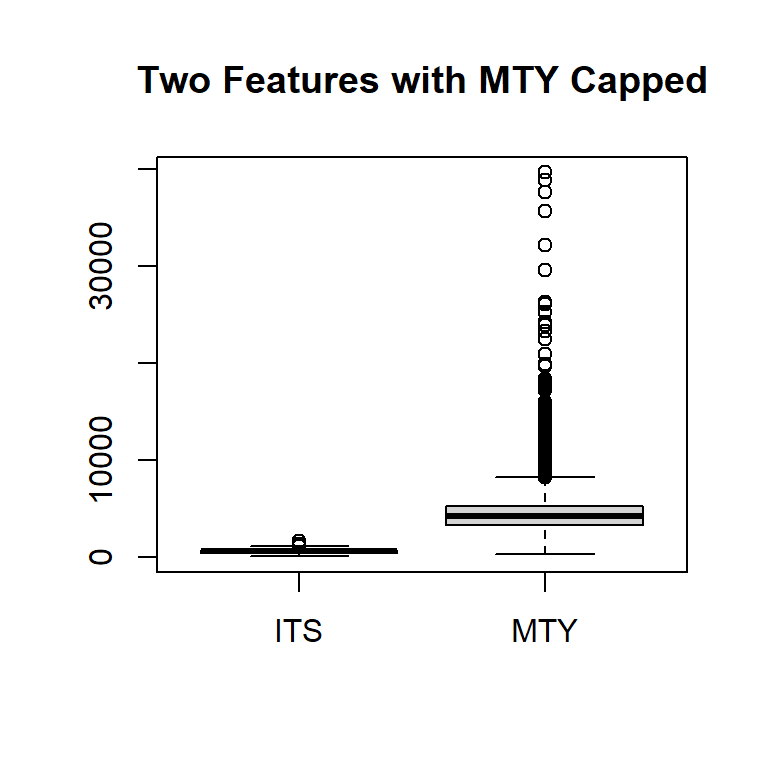``````vioplot(Data[,1],Data[,2])
title('Two Features with MTY Capped')``````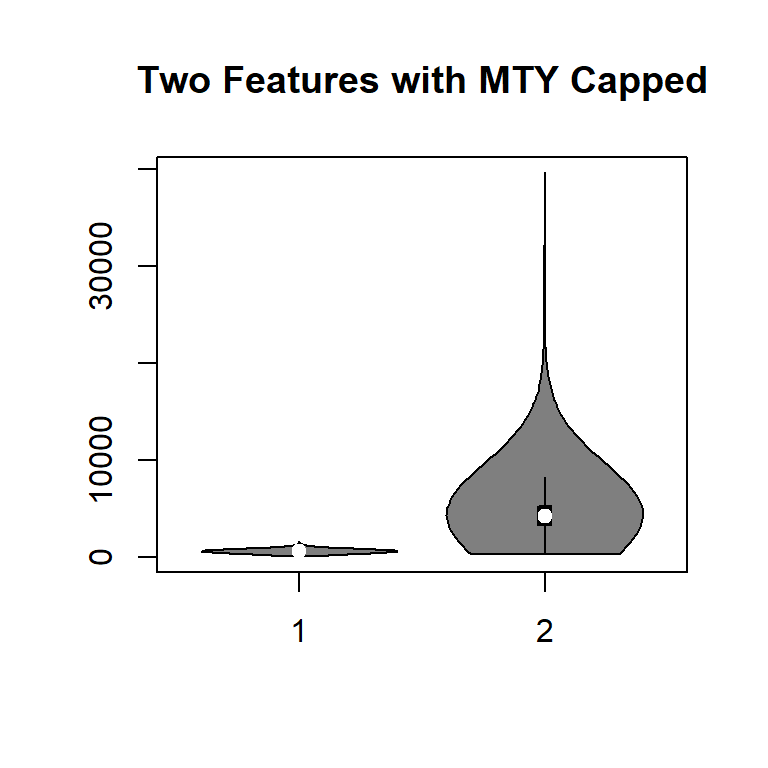## 1.4 Correlation Analysis

Often it is better to visualize the density of scatter plots before calculating correlation coefficients.

``````library(DataVisualizations)
data(ITS)
data(MTY)
Ind2=which(ITS<900&MTY<8000)
if(requireNamespace("ScatterDensity"))
V=DensityScatter(ITS[Ind2],MTY[Ind2],
xlab = 'ITS in EUR',
ylab ='MTY in EUR',
main='Scatter density plot using PDE',
Plotter="native",
DensityEstimation = "PDE")``````